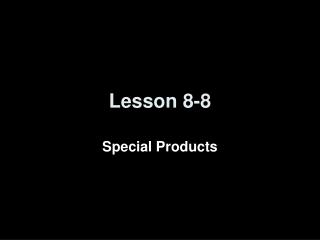DownloadDownload PresentationLesson 8-8

# Lesson 8-8

Download Presentation## Lesson 8-8

- - - - - - - - - - - - - - - - - - - - - - - - - - - E N D - - - - - - - - - - - - - - - - - - - - - - - - - - -
##### Presentation Transcript

1. Lesson 8-8 Special Products

2. Transparency 8 Click the mouse button or press the Space Bar to display the answers.

3. Transparency 8a

4. Objectives • Find the squares of sums and differences • Find the product of a sum and a difference

5. Vocabulary • Difference of squares – two perfect squares separated by a subtraction sign:a2 – b2 = (a + b)(a - b) or (a – b)(a + b).

6. Multiplying Special Polynomials Squares of like polynomials in the following forms,where a and b are constants • Sums: (ax + b)2 • (ax + b)(ax + b) = a2x2 + abx + abx + b2 = a2x2 + 2abx + b2 • Differences: (ax – b)2 • (ax – b)(ax – b) = a2x2 – abx – abx + b2 = a2x2 – 2abx + b2 • One of Each: (ax – b)(ax + b) or (ax + b)(ax – b) • (ax – b)(ax + b) = a2x2 + abx – abx – b2 = a2x2 – b2

7. Square of a Sum F O I L Simplify. Answer: Example 1a Find (7z + 2)2 Check Check your work by using the FOIL method.

8. Square of a Sum Simplify. Answer: Example 1b Find (5q + 9r)2

9. Square of a Difference Square of a Difference Simplify. Simplify. Answer: Answer: Example 2 A. Find (3c – 4)2 B. Find (6e – 6f)2

10. The formula for the area of a square is Area of a square Simplify. Answer: The area of the square is square units. Example 3 Geometry Write an expression that represents the area of a square that has a side length of (2x + 12) units.

11. Product of a Sum and a Difference Answer: Simplify. Example 4a A. Find (9d – 4)(9d + 4)

12. Product of a Sum and a Difference Answer: Simplify. Example 4b B. Find (10g + 13h3)(10g – 13h3)

13. Summary & Homework • Summary: • Square of a Sum (a + b)^2 = a^2 + 2ab + b^2 • Square of a Difference (a- b)^2 = a62 – 2ab - b^2 • Product of a Sum and a Difference (a-b)(a=b) = (a+b)(a-b) = a^2 +b^2 • Homework: • pg. 462 14-48 even